Ex 5.2

Chapter 5 Class 10 Arithmetic Progressions (Term 2)
Serial order wise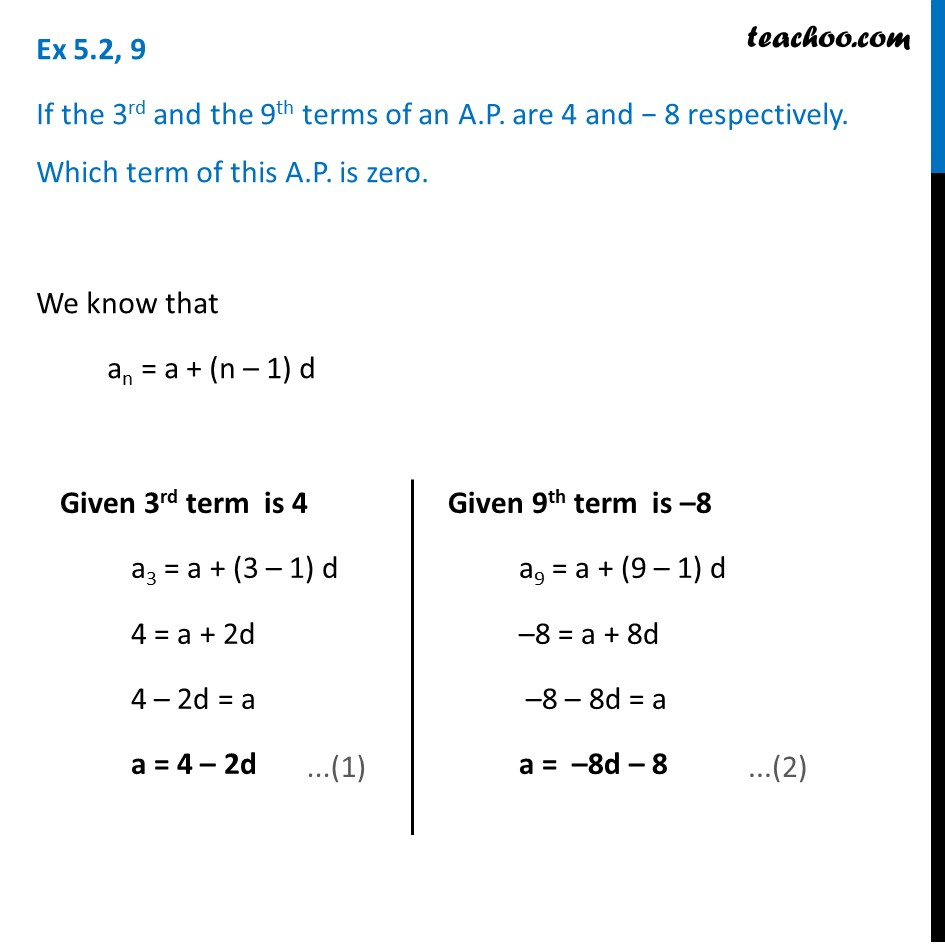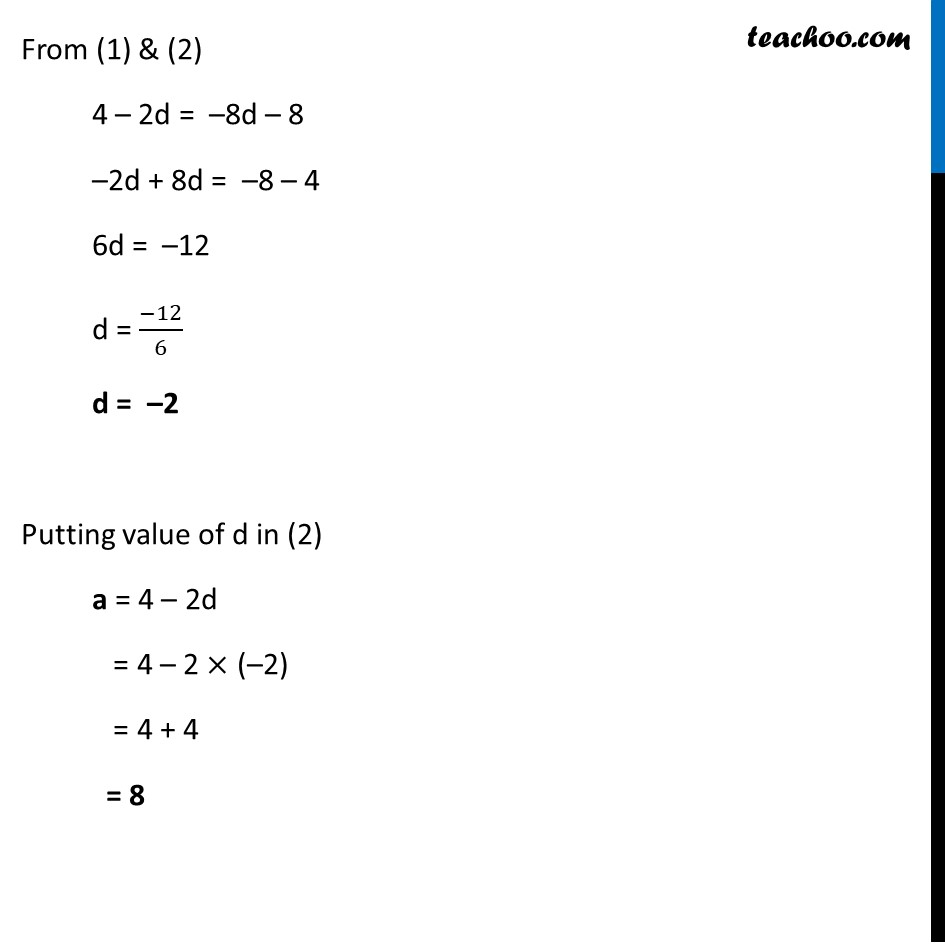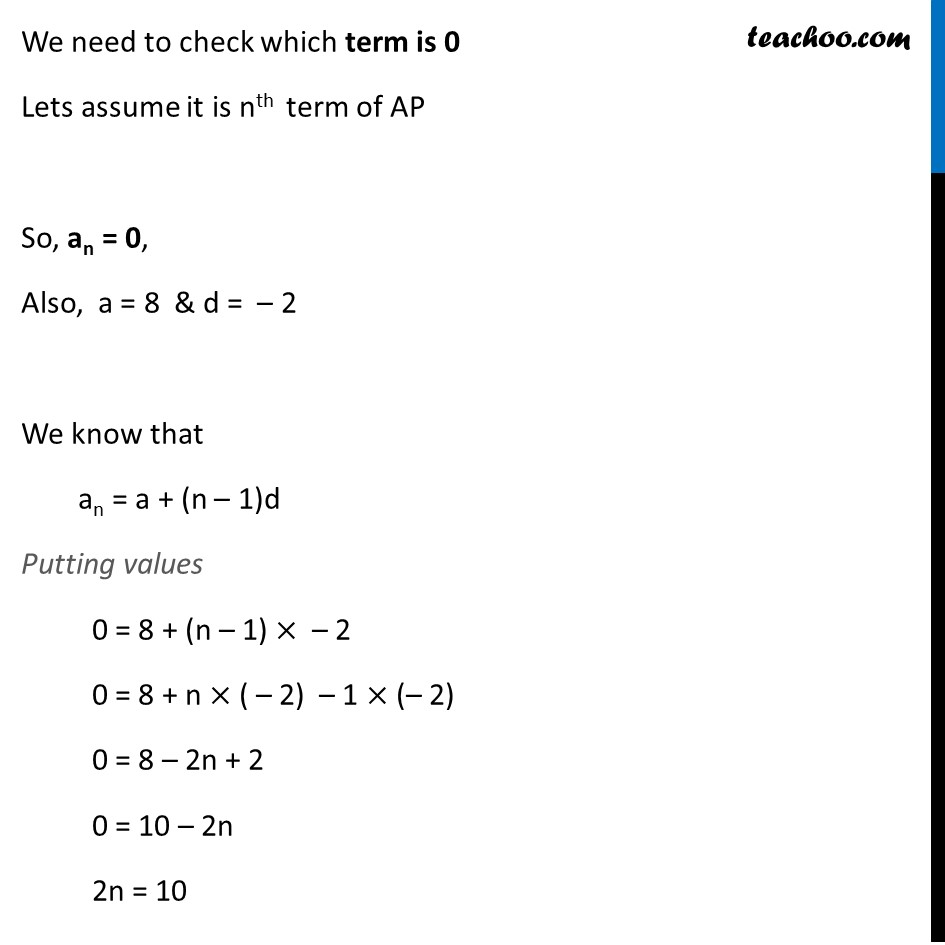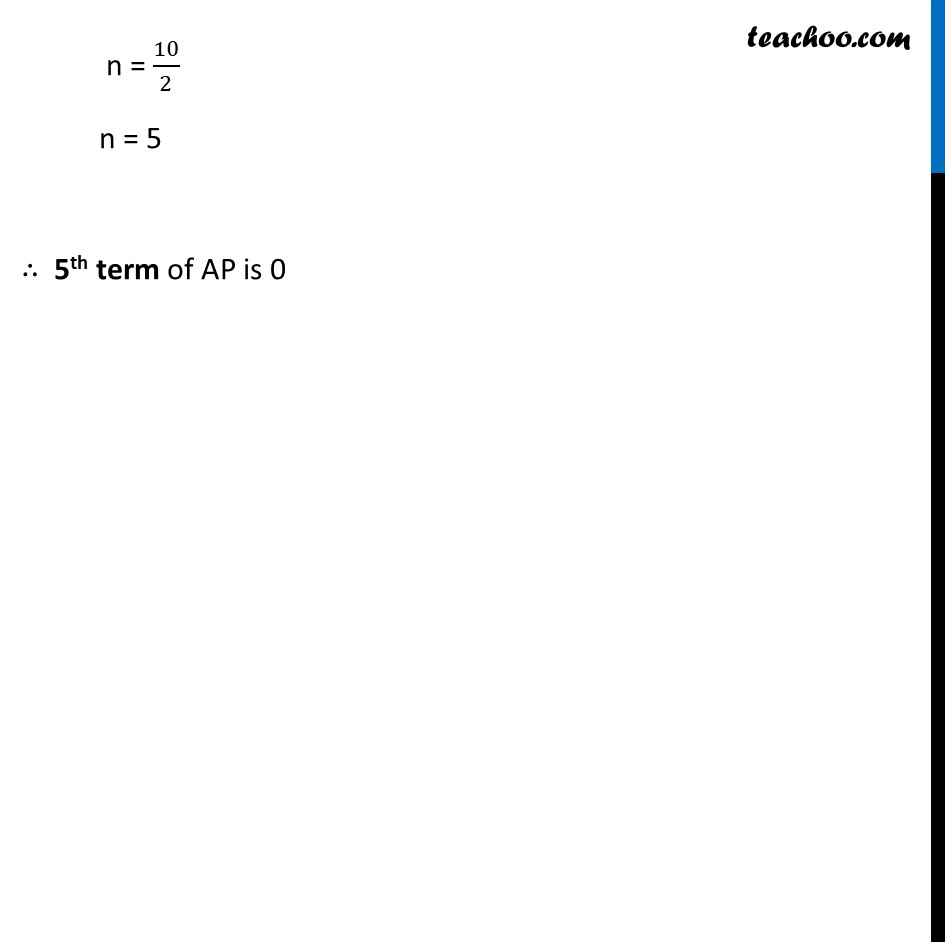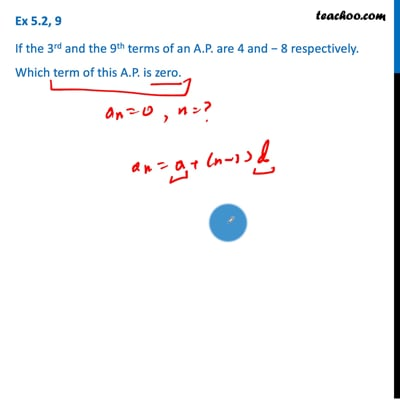This video is only available for Teachoo black users

### Transcript

Ex 5.2, 9 If the 3rd and the 9th terms of an A.P. are 4 and − 8 respectively. Which term of this A.P. is zero. We know that an = a + (n – 1) d Given 3rd term is 4 a3 = a + (3 – 1) d 4 = a + 2d 4 – 2d = a a = 4 – 2d Given 9th term is –8 a9 = a + (9 – 1) d –8 = a + 8d –8 – 8d = a a = –8d – 8 From (1) & (2) 4 – 2d = –8d – 8 –2d + 8d = –8 – 4 6d = –12 d = (−12)/6 d = –2 Putting value of d in (2) a = 4 – 2d = 4 – 2 × (–2) = 4 + 4 = 8 We need to check which term is 0 Lets assume it is nth term of AP So, an = 0, Also, a = 8 & d = – 2 We know that an = a + (n – 1)d Putting values 0 = 8 + (n – 1) × – 2 0 = 8 + n × ( – 2) – 1 × (– 2) 0 = 8 – 2n + 2 0 = 10 – 2n 2n = 10 n = 10/2 n = 5 ∴ 5th term of AP is 0